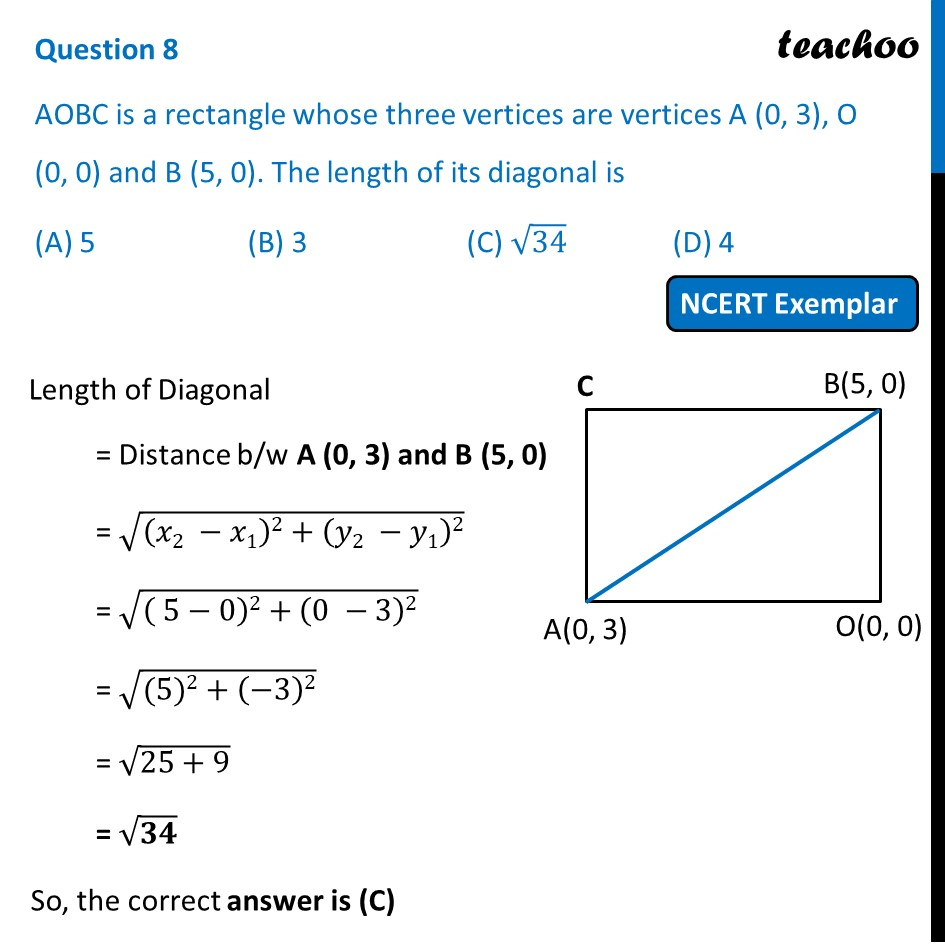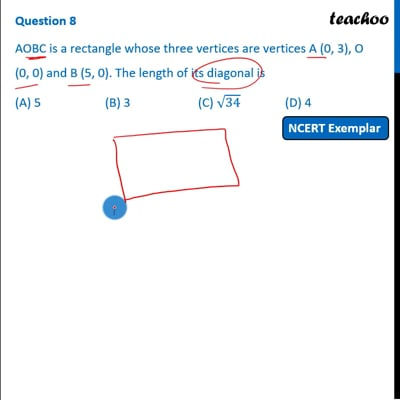NCERT Exemplar - MCQ

Chapter 7 Class 10 Coordinate Geometry
Serial order wise

## (A) 5   (B) 3  (C) √34   (D) 4This video is only available for Teachoo black users

Introducing your new favourite teacher - Teachoo Black, at only ₹83 per month

### Transcript

Question 8 AOBC is a rectangle whose three vertices are vertices A (0, 3), O (0, 0) and B (5, 0). The length of its diagonal is (A) 5 (B) 3 (C) √34 (D) 4 Length of Diagonal = Distance b/w A (0, 3) and B (5, 0) = √((𝑥2 −𝑥1)2+(𝑦2 −𝑦1)2) = √(( 5−0)2+(0 −3)2) = √((5)2+(−3)2) = √(25+9) = √𝟑𝟒 So, the correct answer is (C)